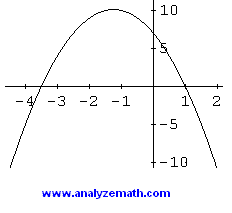# How to Find Zeros of a Function

How to find the zeros of functions; tutorial with examples and detailed solutions. The zeros of a function f are found by solving the equation f(x) = 0.

### Example 1

Find the zero of the linear function f is given by
f(x) = -2 x + 4

### Solution to Example 1

To find the zeros of function f, solve the equation
f(x) = -2x + 4 = 0
Hence the zero of f is give by
x = 2

### Example 2

Find the zeros of the quadratic function f is given by
f(x) = -2 x 2 - 5 x + 7

### Solution to Example 2

Solve f(x) = 0
f(x) = -2 x 2 - 5 x + 7 = 0
Factor the expression -2 x 2 - 6 x + 8
(-2x - 7)(x - 1) = 0
and solve for x
x = -7 / 2 and x = 1
The graph of function f is shown below. The zeros of a function are the x coordinates of the x intercepts of the graph of f.### Example 3

Find the zeros of the sine function f is given by
f(x) = sin(x) - 1 / 2

### Solution to Example 3

Solve f(x) = 0
sin (x) - 1 / 2 = 0
Rewrite as follows
sin (x) = 1 / 2
The above equation is a trigonometric equation and has an infinite number of solutions given by
x = ? / 6 + 2 k ? and x = 5 ? / 6 + 2 k ? where k is any integer taking the values 0 , 1, -1, 2, -2 ...
The graph of f is shown below. The number of zeros of function f defined by f(x) = sin(x) - 1 / 2 are is infinite simply because function f is periodic.### Example 4

Find the zeros of the logarithmic function f is given by
f(x) = ln (x - 3) - 2

### Solution to Example 4

Solve f(x) = 0
ln (x - 3) - 2 = 0
Rewrite as follows
ln (x - 3) = 2
Rewrite the above equation changing it from logarithmic to exponential form
x - 3 = e 2
and solve to find one zero
x = 3 + e 2

### Example 5

Find the zeros of the exponential function f is given by
f(x) = ex2 - 2 - 3

### Solution to Example 5

Solve f(x) = 0
ex2 - 2 - 3 = 0
Rewrite the above equation as follows
ex2 - 2 = 3
Rewrite the above equation changing it from exponential to logarithmic form
x2 - 2 = ln (3)
Solve the above equation to find two zeros of f
x1 = square root [ln (3) + 2]
and
x2 = - square root [ln (3) + 2]